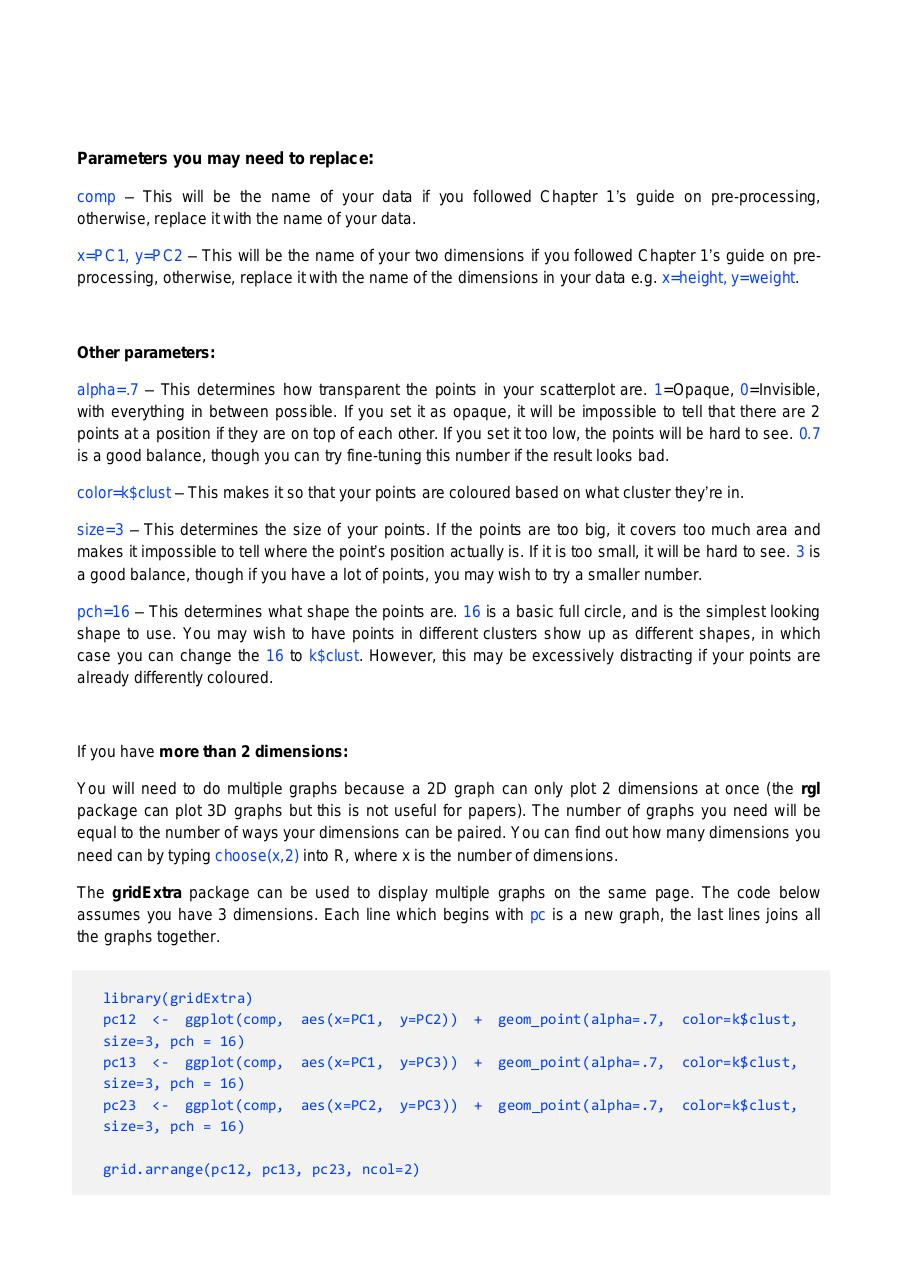# PDF Archive

Easily share your PDF documents with your contacts, on the Web and Social Networks.

## 2B R Code.pdfPage 1 2 3 456102

#### Text preview

Parameters you may need to replace:
comp – This will be the name of your data if you followed Chapter 1’s guide on pre-processing,
otherwise, replace it with the name of your data.
x=PC1, y=PC2 – This will be the name of your two dimensions if you followed Chapter 1’s guide on preprocessing, otherwise, replace it with the name of the dimensions in your data e.g. x=height, y=weight.

Other parameters:
alpha=.7 – This determines how transparent the points in your scatterplot are. 1=Opaque, 0=Invisible,
with everything in between possible. If you set it as opaque, it will be impossible to tell that there are 2
points at a position if they are on top of each other. If you set it too low, the points will be hard to see. 0.7
is a good balance, though you can try fine-tuning this number if the result looks bad.
color=k\$clust – This makes it so that your points are coloured based on what cluster they’re in.
size=3 – This determines the size of your points. If the points are too big, it covers too much area and
makes it impossible to tell where the point’s position actually is. If it is too small, it will be hard to see. 3 is
a good balance, though if you have a lot of points, you may wish to try a smaller number.
pch=16 – This determines what shape the points are. 16 is a basic full circle, and is the simplest looking
shape to use. You may wish to have points in different clusters show up as different shapes, in which
case you can change the 16 to k\$clust. However, this may be excessively distracting if your points are

If you have more than 2 dimensions:
You will need to do multiple graphs because a 2D graph can only plot 2 dimensions at once (the rgl
package can plot 3D graphs but this is not useful for papers). The number of graphs you need will be
equal to the number of ways your dimensions can be paired. You can find out how many dimensions you
need can by typing choose(x,2) into R, where x is the number of dimensions.
The gridExtra package can be used to display multiple graphs on the same page. The code below
assumes you have 3 dimensions. Each line which begins with pc is a new graph, the last lines joins all
the graphs together.

library(gridExtra)
pc12 &lt;- ggplot(comp,
size=3, pch = 16)
pc13 &lt;- ggplot(comp,
size=3, pch = 16)
pc23 &lt;- ggplot(comp,
size=3, pch = 16)

aes(x=PC1,

y=PC2))

+

geom_point(alpha=.7,

color=k\$clust,

aes(x=PC1,

y=PC3))

+

geom_point(alpha=.7,

color=k\$clust,

aes(x=PC2,

y=PC3))

+

geom_point(alpha=.7,

color=k\$clust,

grid.arrange(pc12, pc13, pc23, ncol=2)